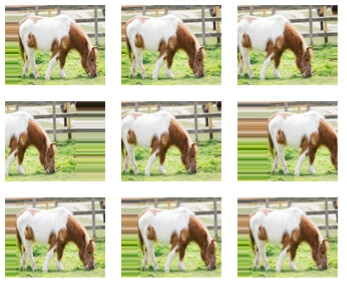# How to Shift image(data augmentation)

I want to implement the data augmentation like below image.
I found the similar topic, however I could not find the solutions by using kornia
https://discuss.pytorch.org/t/is-there-any-function-to-shift-image-by-x-axis-or-y-asix/80838

Is there any data augmentation method to shift image by x axis or y asix?Something like this ?

``````import torch
import kornia
import numpy as np
from PIL import Image
import matplotlib.pyplot as plt

img = np.array(Image.open('pepper.png'))

img_tensor = torch.tensor(img.transpose([2, 0, 1])).float()

fig = plt.figure(figsize=(15, 15))
for i in range(4):
for j in range(4):
ax = plt.subplot(4, 4, i*4 + j +1)
img_translated = kornia.random_affine(
img_tensor,
degrees=0,
translate=(0.3, 0.3)
)
ax.imshow(img_translated.squeeze().permute(1, 2, 0).byte())
``````

Output:

2 Likes

Thanks for quickly response.
Can I change shift mode from ‘zeros’ to ‘nearest’ as shown in below image?That function does not seem to have padding_mode, but one can instantiate a RandomAffine class to get the padding mode like this

``````affine = kornia.augmentation.RandomAffine(degrees=0, translate=(0.3, 0.3), padding_mode='border')
fig = plt.figure(figsize=(15, 15))
for i in range(4):
for j in range(4):
ax = plt.subplot(4, 4, i*4 + j +1)
img_translated = affine(img_tensor)
ax.imshow(img_translated.squeeze().permute(1, 2, 0).byte())
``````
1 Like